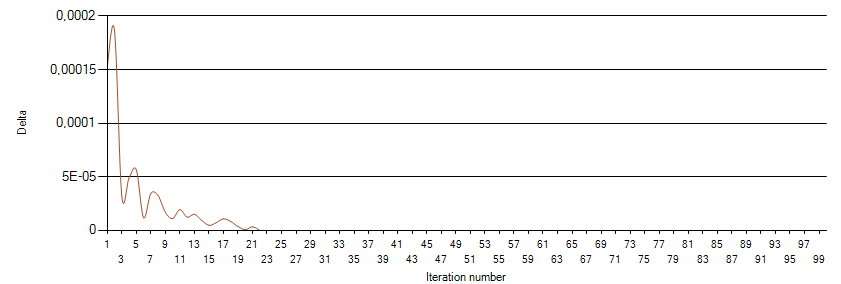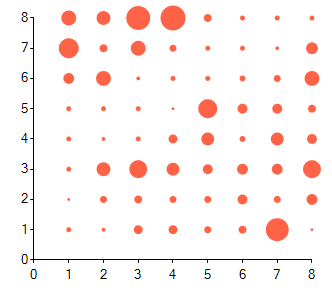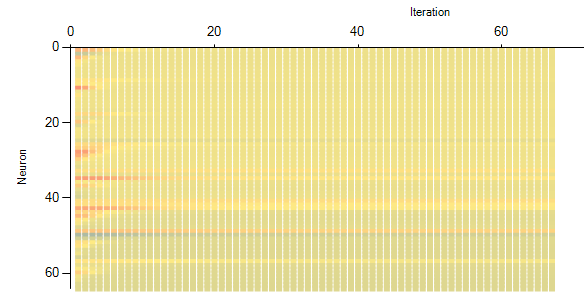# Figure 10 - Neuron map of the Iris dataset

 Figure 10 - Neuron map of the Iris dataset Delta decreases as the number of iterations increases and the changes in weights become smaller. This is represented on the weights change ratio per iterations chart. The value of delta is plotted against iteration numbers to show how it decreases over time. A sample of the program output is shown in Figure 11. Of note is that the following five charts are updated as the training process is happening. This makes for an interactive presentation of the training process.Figure 11 - Weight change ratio whil training the Iris data set Some nodes are selected as the BMU more often than others. This is shown on the best matching units graph. The number of wins can be configured to be represented by the size of the node’s dot, or explicitly written as a number. A sample of the program’s output can be seen in Figure 12.Figure 12 - Best matching units found while training on the Iris dataset Neuron heatmap shows the average change of a neuron’s weight per iteration. Once the heatmap starts showing distinct lines, the training process is nearly finished. An example of the program’s output can be seen on Figure 13.Share with your friends: